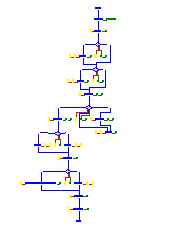# Annotated Control Flow Graph## Description

This is a control flow graph as used as data structure in a compiler. The yellow nodes of this graph represent the assignments, the pink rhomb nodes are the branch statements, and the aquamarine ellipse nodes are the entry and exit nodes of the represented program. The control flow is drawn by blue edges. The dark red nodes with yellow border are the constant informations as constructed by a constant propagation algorithm. The dark green nodes are the live variables as calculated by a liveness analysis.

The partitioning algorithm in this layout is depth first search. Edges are drawn by orthogonal edge segments (manhattan layout). The branches are specified by bent near edges where the edge label is drawn at the bend point. The lists of annotations are formed by near edges (except at the branch nodes, because this would be too confusing).

The blue control flow graph has edge class 1. The lists of constant informations are formed by edges of class 2, and the lists of liveness informations are formed by edges of class 3. Thus, it is possible to hide these lists interactively by selecting the operation Hide edge class 2 and 3 . Select here to see the result.

Last change: 21.4.1995 Georg Sander / sander@cs.uni-sb.de